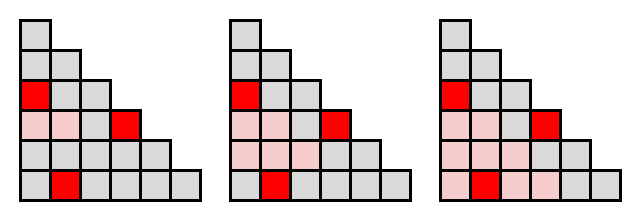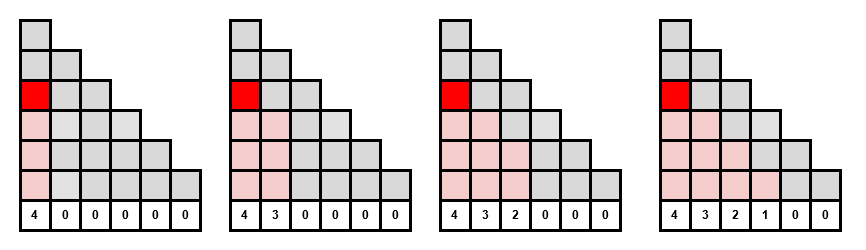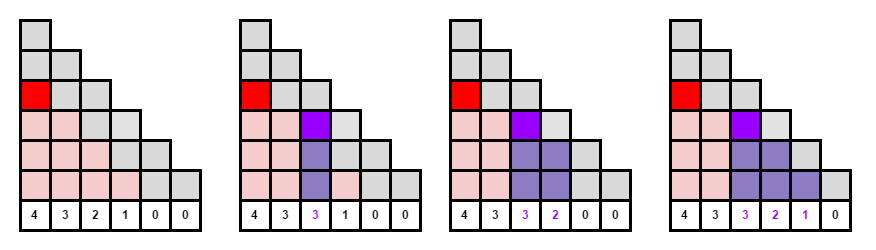2016-CCOQR-Q3 Data Structure

# 2016-CCOQR-Q3 Data Structure

Problem Description
View Problem

## Key Insight #1

One of the first things to notice is that although the problem is presented as a pyramid it does not have to be solved using this awkward data structure. The same problem can be represented using a 2D array where a block that requires support needs the block immediately underneath it and the block underneath to the right to contain data.## Analysis

The problem has a very simple solution where you allocate a 2D boolean array and for each input point a one is placed in the array at the start point and then at the next row down two ones are placed and then 3 ones are placed, etc.The problem with this approach is simply that this would require too much memory and too much time. The problem set included a possible 1 BILLION rows requiring a total of 800 exabytes if each cell was stored as a byte. Obviously processing all the input points with an array that size would not be possible on any machine.

Brute Force Implementation

A brute force solution that receives 6/15 points and uses a recursive dynamic programming approach

View Brute Force Solution

## Key Insight #2

The way to reduce the complexity of the problem is to break the problem into distinct columns and determine the number of rows from the bottom of the diagram that would be impacted by adding a new data point.

So you create an array the size of the bottom row and initialize it with 0. You then start at the first column impacted by the point and fill the array with a count of rows filled in above it. You then move to the right and repeat the process until your row count is 0.The really important aspect of this process is that for the next data point the process is identical and except that instead of setting the array value you compute the maximum of the previous value and the one introduced now. This deals with the problem of multiple data points impacting the same area:The final step is simply to sum up all the counts in the array.

## Key Insight #3

You don’t need an array to add the numbers computed above. If the point array is sorted, you can compute the total sum with a single loop over the columns by remembering what the previous row count was. This approach requires the cost of sorting the array O(N Log(N)) and a single pass through the columns O(N).

Please attempt to solve the problem on your own before viewing the solution!
View Solution